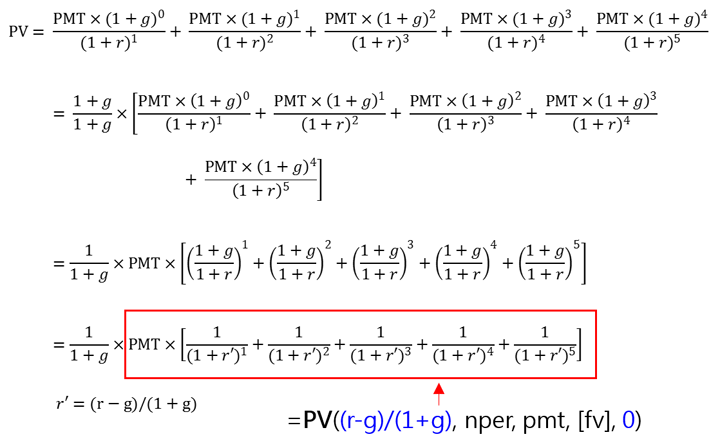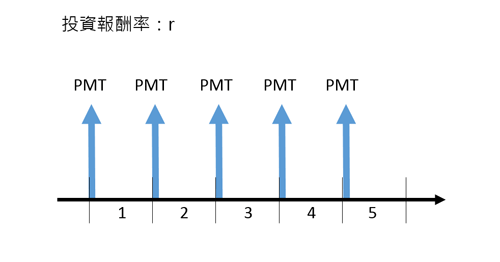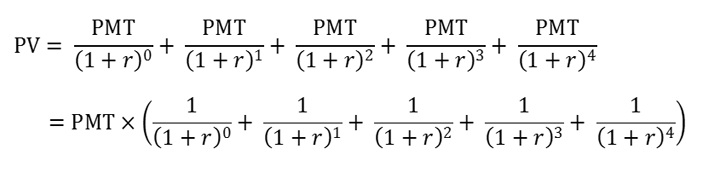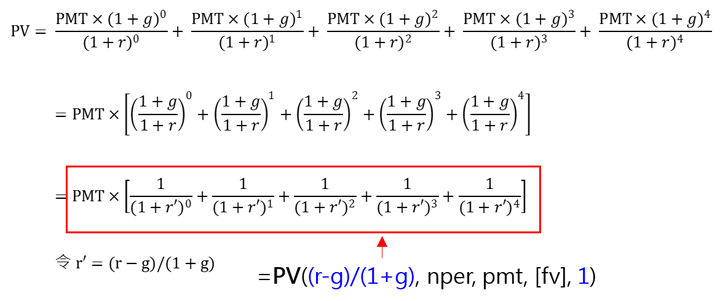# 用Excel計算成長型年金

﻿

Excel 的FV及PV財務函數，是以輸入之參數描述現金流量，每種參數都只有一個，對pv或fv單筆金額的參數不會有問題，但pmt參數因為屬於年金，每一期都有現金流量，所以每一期的pmt都必須一樣才適用。而且，pmt參數和nper(期數)及type參數一起才能完整描述現金流量。

pmt=10000、nper=5、type=0

 函數 期末(type=0) 期初(type=1) 備註 PV =PV((r-g)/(1+g), nper, pmt)/(1+g) =PV((r-g)/(1+g), nper, pmt, 0, 1) fv=0 FV =FV((r-g)/(1+g), nper, pmt) *(1+g)^(nper-1) =FV((r-g)/(1+g), nper, pmt, 0, 1) *(1+g)^nper pv=0

## 範例一：退休金

Peter預計60歲退休，退休後生活費預估每年60萬元，假設退休後報酬率每年6.0%，通貨膨漲率2.0%，要準備多少退休金才足以使用至100歲？(參考投影片http://goo.gl/4KqNhK第3~4頁)

=PV((6%-2%)/(1+2%), 40, 600000)/(1+2%)
=-1,177萬9,943元 (負值代表現金流出)

=PV((6%-2%)/(1+2%), 40, 600000*(1+2%)^0.5)/(1+2%)
=-1,189萬7,160元 (負值代表現金流出)

## 範例二：定期不定額投資

=FV((10%-3%)/(1+3%),10,-120000)*(1+3%)^9
=214萬2,559元

## 公式推導

### PV函數：期末年金Excel的PV函數對期末年金PMT(type=0)的計算公式如下：### PV函數：期初年金Excel的PV函數對期初年金PMT(type=1)的計算公式如下：Posted by 怪老子理財 on 2015年4月7日
﻿

■ 本網站內容儘可能精確完整，但不保證無誤。若做為投資依據，風險請自行斟酌 ，本網站不負賠償之責任。
■ 網站所有資料均為版權所有，非經書面允許請勿轉載或使用。